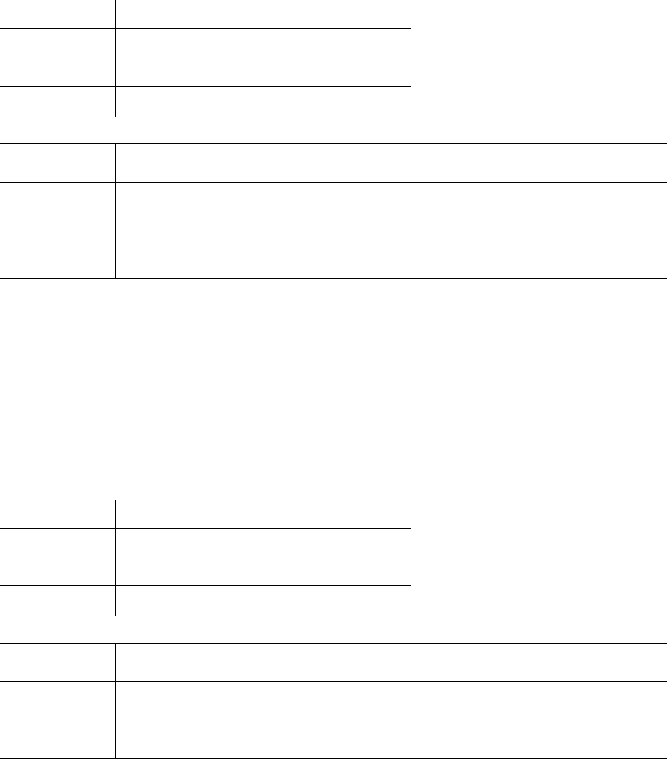# ECO 311 Miami U eco311FinalFall 16Fall 1

21 views16 pages
Eco311, Final Exam, Fall 2016.
Prof. Bill Even
1
Did you agree to take the optional portion of the final exam Yes No
Directions
There are two parts to the final exam. Everyone must take the first part of the exam which includes 20
questions. Students who elected to take the optional portion of the final exam are required or allowed to
complete the second part. For those who elected to take the optional portion of the final exam, 1/3 of
the overall score for the exam will be based on that portion of the exam.
Place all answers in the space provided below or within each question. Round all numerical answers to
the nearest 100th (e.g. 1.23) unless told otherwise. Each question is worth 3 points unless otherwise
indicated.
The formula sheet and tables with the standard normal CDF, the F- and Chi-squared distributions are
attached to the end of the exam.
Unlock document

This preview shows pages 1-3 of the document.
Unlock all 16 pages and 3 million more documents.Eco311, Final Exam, Fall 2016.
Prof. Bill Even
2
A regression analysis of the loans received by applicants (loanamt measured in 1000s of \$) is provided
below. The control variables include the applicant’s annual income (appinc), dummy variables for
whether the applicant was a single male (sgl_male) or single female (sgl_female). Married applicants
are the reference group.
You should be able to interpret the second regression below based on the Stata commands that follow
the first regression.
1. Based on the above regressions, the value of the Chi-squared test statistic for the null hypothesis
that the residuals in the loan equation are homoscedastic is ____________ and the test statistic
has a Chi-squared distribution with _________ degrees of freedom.
2. The null hypothesis that the residuals in the loan equation are homoskedastic is rejected at the .01
level if the chi-squared statistic is (greater, less) than _________.
_cons -33162.71 4496.584 -7.38 0.000 -41981.28 -24344.14
yhat2 -.0688413 .1024685 -0.67 0.502 -.2697995 .1321169
yhat 277.7028 46.11293 6.02 0.000 187.2675 368.1381
ehat2 Coef. Std. Err. t P>|t| [95% Conf. Interval]
Total 8.8117e+11 1,970 447296080 Root MSE = 19080
Residual 7.1647e+11 1,968 364058674 R-squared = 0.1869
Model 1.6471e+11 2 8.2353e+10 Prob > F = 0.0000
F(2, 1968) = 226.21
Source SS df MS Number of obs = 1,971
. reg ehat2 yhat yhat2
. gen yhat2=yhat^2
. predict yhat, xb
. gen ehat2=ehat^2
. predict ehat, residual
_cons 118.8811 2.619157 45.39 0.000 113.7445 124.0177
sgl_female -31.18393 4.973165 -6.27 0.000 -40.93716 -21.43071
sgl_male -19.72992 4.048456 -4.87 0.000 -27.66964 -11.79021
appinc .3858079 .0186439 20.69 0.000 .349244 .4223719
loanamt Coef. Std. Err. t P>|t| [95% Conf. Interval]
Total 12856381 1,970 6526.08172 Root MSE = 71.923
Residual 10175210.8 1,967 5172.95921 R-squared = 0.2085
Model 2681170.23 3 893723.412 Prob > F = 0.0000
F(3, 1967) = 172.77
Source SS df MS Number of obs = 1,971
. reg loanamt appinc sgl_*
Unlock document

This preview shows pages 1-3 of the document.
Unlock all 16 pages and 3 million more documents.

Eco311, Final Exam, Fall 2016.
Prof. Bill Even
3
3. If you wanted to perform a Breusch-Pagan test for heteroskedasticity in the above loan regression,
what regression command would you execute in Stata? (e.g. reg y x use the relevant variable
names from above to define what variables would be used for y and x and define any additional
variables that are required).
4. Based on the above regressions, we can conclude that the variance of the residuals in the loan
equation
a. Is always greater for those with larger predicted loan amounts
b. Is always less for those with larger predicted loan amounts
c. Initially rises with predicted loan amounts, but eventually falls
d. Initially falls with predicted loan amounts, but eventually rises
5. If the loan equation has heteroskedasticity,
a. The standard OLS estimates of the coefficients are biased
b. The standard OLS estimates of the standard errors for the coefficients are incorrect
c. The standard OLS estimates of the coefficients are no longer efficient
d. All of the above
e. Only b and c
6. Suppose that you use Stata to estimate the above loan regression with OLS and then you choose
to estimate robust standard errors. Compared to conventional OLS, the use of robust standard
errors will
a. Not cause any change in the estimated coefficients
b. Will cause all the standard errors to rise
c. Will cause all the t-statistics to shrink (in absolute value)
d. All of the above
e. None of the above
7. Suppose that you want to estimate a linear probability model with weighted least squares. If p is
the predicted probability of a yes obtained from the LPM, what is the weight that should be
attached to each observation?
Unlock document

This preview shows pages 1-3 of the document.
Unlock all 16 pages and 3 million more documents.